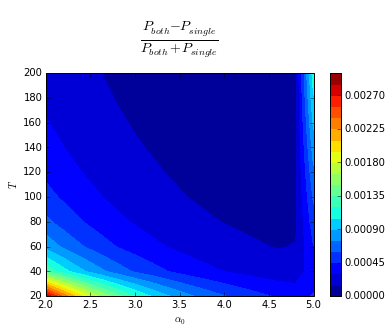# Holonomic quantum computing with cat-codes

See arXiv 1503.00194.

Each column in the table corresponds to a different gate. Just start by playing the animations.

The dissipation coefficient is set to 1 in all simulations bellow. Therefore the time is dimensionless.

Two Blobs Three Blobs
Phase Gate Population Gate Phase Gate 2-Population Gate 3-Population Gate
Description The right blob goes on a circle. The two blobs collide. The right blob goes on a circle. Two of the three blobs collide. The three blobs collide.
Initial State $$\left| \alpha_0 \right\rangle + \left| -\alpha_0 \right\rangle$$ $$\left| \alpha_0 \right\rangle$$ $$\left| \alpha_0 \right\rangle + \left| \scriptstyle\sqrt{-1} \displaystyle\alpha_0 \right\rangle + \left| \scriptstyle\sqrt{-1}^2 \displaystyle\alpha_0 \right\rangle$$ $$\left| \alpha_0 \right\rangle + 2\left| \scriptstyle\sqrt{-1} \displaystyle\alpha_0 \right\rangle$$ $$\left| \alpha_0 \right\rangle$$
Animation for $$\alpha_0=2$$
Scaling of the Purity wrt $$\alpha_0$$ and $$T$$

$$1-P\propto\alpha_0^{-1.815}T^{-0.976}$$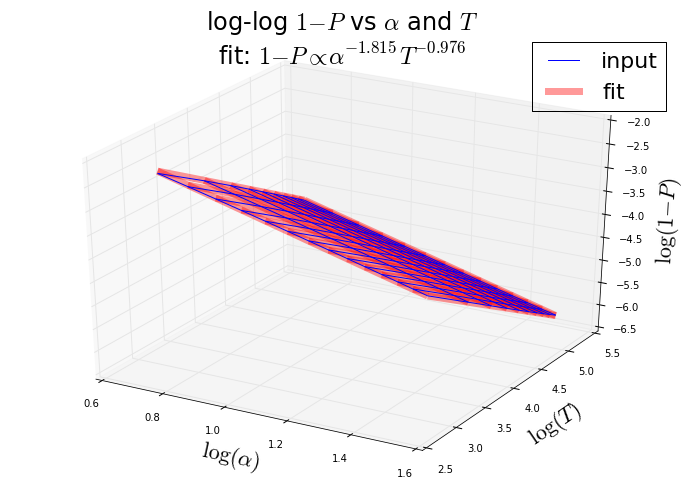$$1-P\propto\alpha_0^{-3.945}T^{-0.984}$$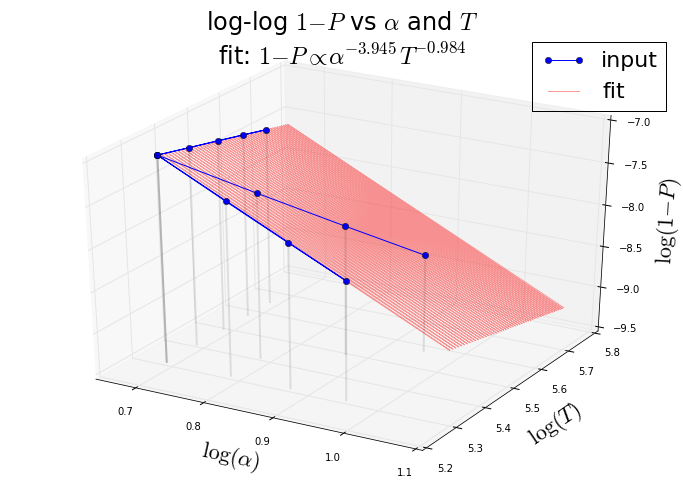$$1-P\propto\alpha_0^{0.967}T^{-0.988}$$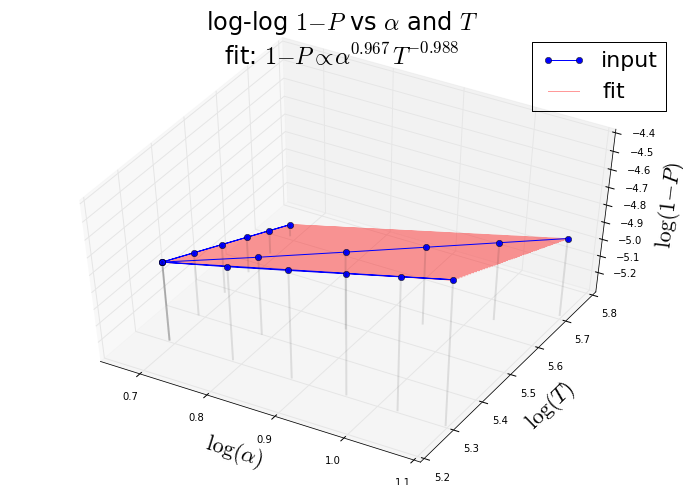Scaling of the Purity wrt $$\alpha_0$$ and $$v$$(the phase-space "speed" of the blobs)

$$1-P\propto\alpha_0^{-1.815}v^{0.976}$$

$$v\propto 1/T$$

$$1-P\propto\alpha_0^{-3.945}v^{0.984}$$

$$v\propto 1/T$$

$$1-P\propto\alpha_0^{-0.020}v^{0.988}$$

$$v\propto\alpha_0/T$$

The plots from the animation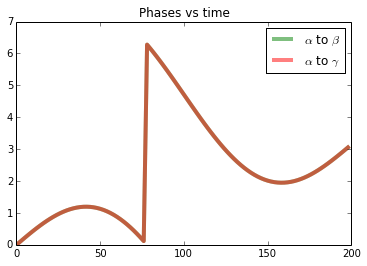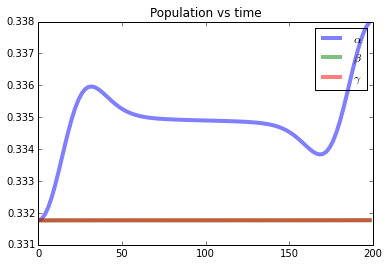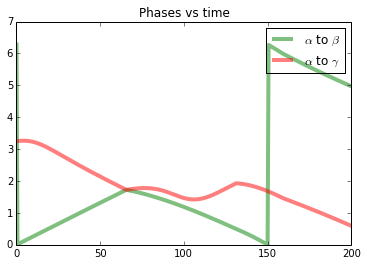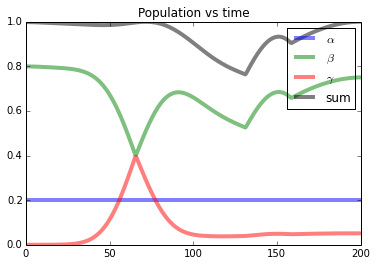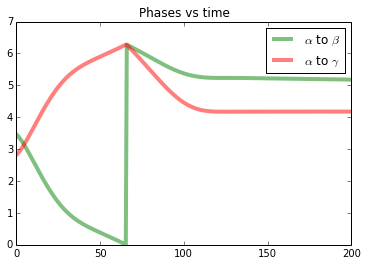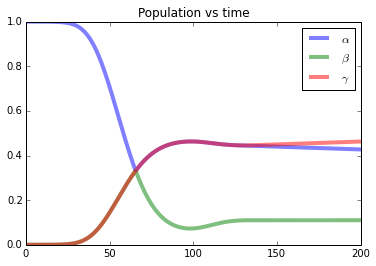Other remarks Only the area matters for the induced phase. The loss in purity also seems to scale with the area, given that the results for a single circle of area A and for two circles each of area A/2 were the same (the total area was the same, the path taken was √2 bigger). See the next table. For a fixed $$\alpha_0$$ the worst imperfections induced during the evolution are closer to the center. Strange artefacts for the outer most blob, maybe due to cutoff dimension being too low. For a fixed $$\alpha_0$$ the imperfections are induces mainly on the way into and out of the collision (not during the collision itself).
Technical Details Various, see the code. 15000 samples, N=80 cutoff for the (1-P) log log plot. 20000 samples, N=40 for the comparisons in the next table, 15000 samples, N=40 for the animation. 60000 samples, N=40 cutoff 200000 samples, N=55 cutoff (which is insufficient for $$\alpha_0 \gt 2.6$$) 680000 samples, N=55 cutoff (possibly insufficient for these plots and animations) 680000 samples, N=55 cutoff
Computer Code Files phase gate, population gate, animation visualization, simulation visualization, simulation visualization, simulation

Comparing the case of one and two moving blobs for the 2-blob phase gate. See the first column of the previous table (which corresponds to the first column of this table).
Two Blobs, Only the Right One Moving Two Blobs Moving in Opposite Directions
Plots and animations for various radiuses of the circles.
Description The right blob goes on a circle. Both blobs go on circles. The total area of the circles is the same as in the one-blob case.
Scaling of the Purity wrt $$\alpha_0$$ and $$T$$

$$1-P\propto\alpha_0^{-1.815}T^{-0.976}$$$$1-P\propto\alpha_0^{-1.797}T^{-0.974}$$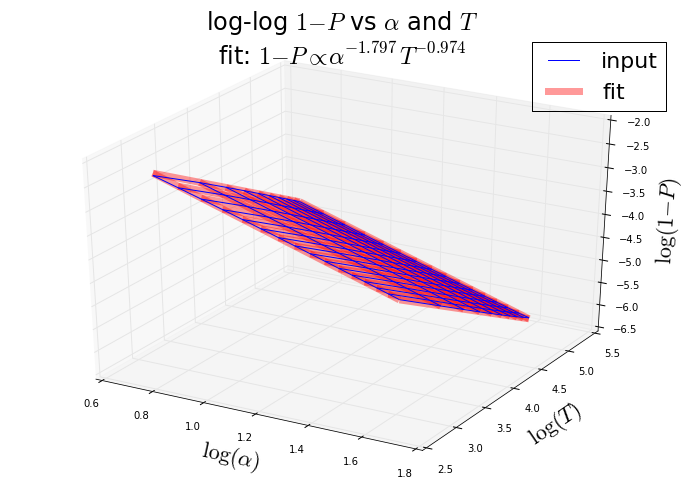The final relative phase between the blobs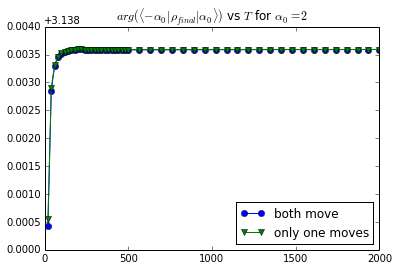Total time T on the x axis

The final purity of the system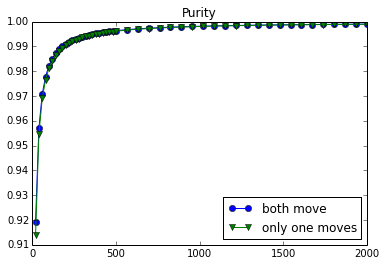Total time T on the x axis

The contrast between the final purities for both approaches